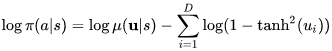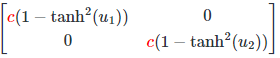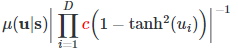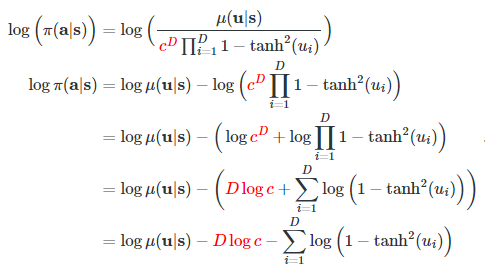# Activation function and distribution for action space between 0 and 1?

Hi,

I am building my first REINFORCE (policy gradient) model with a continuous action space between 0 and 1. Right now, I use the following code for this:

``````        self.loc_layer = nn.Sequential(
nn.Linear(size, n_params),
nn.Sigmoid()
)
self.scale_layer = nn.Sequential(
nn.Linear(size, n_params),
nn.Sigmoid()
)

# ...

loc = self.loc_layer(x)
if self.training:
scale = self.scale_layer(x)
dist = Normal(loc=loc, scale=scale)
params = dist.sample()
log_prob = dist.log_prob(params).sum(dim=1)
params = params.clamp(min=0, max=1)
else:
params = loc
log_prob = None
``````

However, this does not seem right to me. For example, I do not like that I have to clamp to ensure that the actions (`params`) are between 0 and 1. Therefore, I consider using a Beta or Logit-Normal distribution instead. In other examples, I have seen that Softplus is often used as the activation function for the scale argument. But this does not seem right here because I want to limit the action space.

Can anyone with some experience in this area give me a recommendation to which activation function and distribution to use in this case?

Three solutions:

1. use a normal distribution, use tanh as `mu` activation (to keep the center in range, prevent shifting too much) and then clamp, but you should do clamping only on the action sent to the environment, and not actions stored in buffers. In this way, you are not changing the pdf of your action, but changing the reward distribution.

2. use a normal distribution, use tanh to clamp your output right after sampling, but in order to calculate the log probability of your sample, you need to do this step, which is used in the Soft Actor Critic algorithm.It should be relatively easy to deduce this equation

3. use a different distribution, but normally, the normal distribution performs the best in all algorithms, I have not tried Beta or Logit-Normal distribution and don’t know their performance. This method is theoretically possible, but result is not garanteed.

Thanks a lot for your answer! Do you think it’s better to use a transformed tanh (i.e. `(tanh + 1)/2`) instead of sigmoid for actions in the range of 0 to 1? If so, because of the stronger gradient or is there another reason?

I have switched to softplus for the activation function of the scale parameter (since this seems be commonly used) and it seems to work. Is this what you would have recommended as well?

tanh and sigmoid does not quite affect the performance.

Anything that will output a positive value can be used as your sigma (scale) activation, softplus is commonly used because its smooth, close to linear (y=x) when x >> 0 and works on the negative region.

Thanks for your advice, and I have a math question. I know how to transfer a sampled value of a specific normal distribution to a specifc range. 1.using tanh to bound the sampled_value in range [-1,1], 2. use the formula here to transfer it to specific range. But what about bounding the log_prob value of the sampled value to specific range? The formula you referred of SAC, is only suitable when the target range is in [-1,1].

• A transformed Beta distribution policy is an option. See for example in this paper. However, I haven’t personally gotten Beta distributions to work. The training is always very unstable.
• Have the network output actions in [-1, 1] and transform to the desired range as part of the environment. See here.

Long answer regarding the transformation of sampled actions:
In general, doing a location-scale transformation of your clamped actions in [-1, 1] should not affect the SAC entropy correction formula. To see why, consider your transformation of the type l + c*a, where a = tanh(u) just like in the SAC paper (l being a location term, c being a scaling factor).The term in the log behind the sum comes from the derivate of your tanh transformation. If you instead take the derivative of l + c*tanh(u), the term l will immediately fall away and you will haveas the derivative of the tanh-function applied element-wise to your actions.
You then want to take the log of the following densityThe scaling term c will persist, but due to the log you can move it out of the sum.Here D is the dimensionality of your action space.
Now when you want to perform backprop, the term D log c only depends on the constants D and c. These are irrelevant for the derivative.
What does this mean for you?
You can use 6+4*tanh(u) to transform your actions between e.g. [2, 10] without having to change the entropy correction formula.

Source: This has been part of my master’s thesis.

1 Like